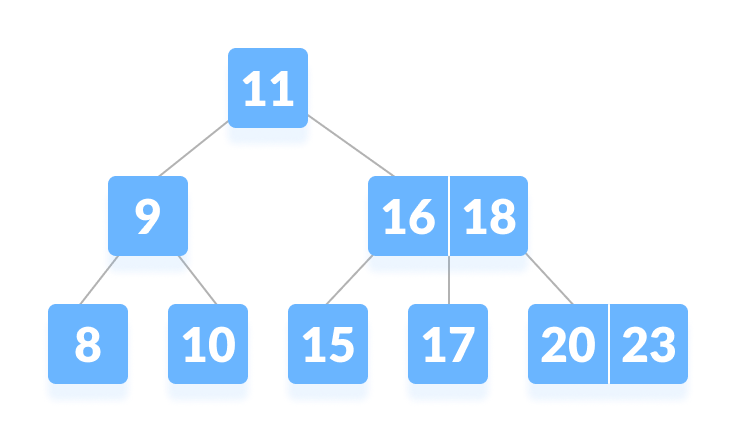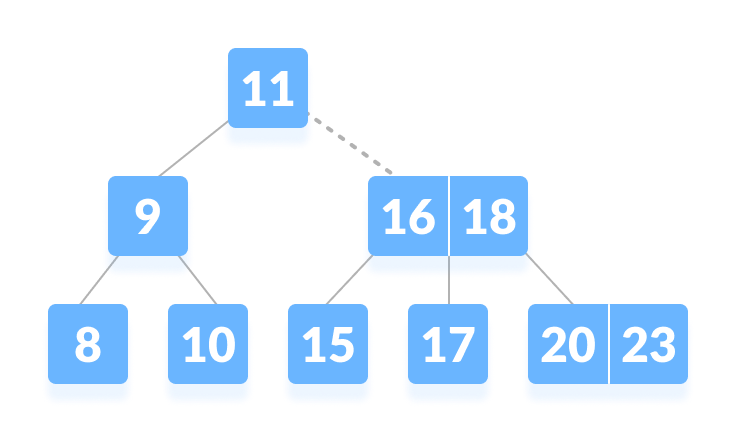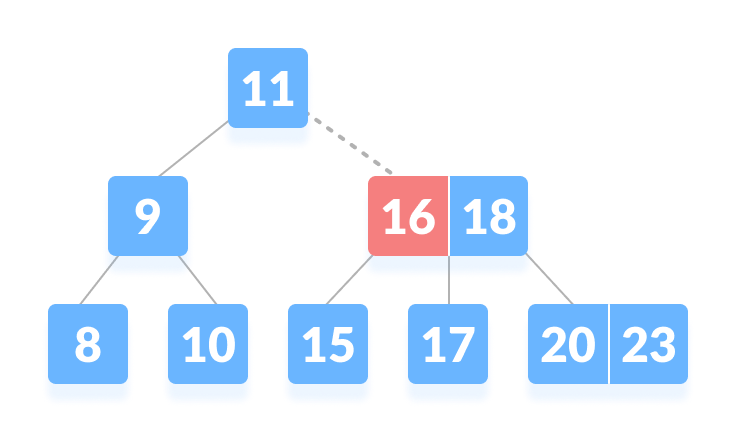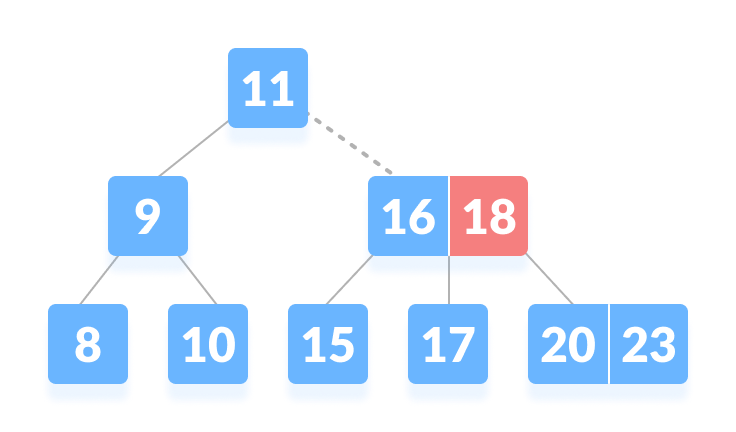# B-tree

In this tutorial, you will learn what a B-tree is. Also, you will find working examples of search operation on a B-tree in C, C++, Java and Python.

B-tree is a special type of self-balancing search tree in which each node can contain more than one key and can have more than two children. It is a generalized form of the binary search tree.

It is also known as a height-balanced m-way tree.

## Why do you need a B-tree data strcuture?

The need for B-tree arose with the rise in the need for lesser time in accessing the physical storage media like a hard disk. The secondary storage devices are slower with a larger capacity. There was a need for such types of data structures that minimize the disk accesses.

Other data structures such as a binary search tree, avl tree, red-black tree, etc can store only one key in one node. If you have to store a large number of keys, then the height of such trees becomes very large and the access time increases.

However, B-tree can store many keys in a single node and can have multiple child nodes. This decreases the height significantly allowing faster disk accesses.

## B-tree Properties

1. For each node x, the keys are stored in increasing order.
2. In each node, there is a boolean value x.leaf which is true if x is a leaf.
3. If n is the order of the tree, each internal node can contain at most n - 1 keys along with a pointer to each child.
4. Each node except root can have at most n children and at least n/2 children.
5. All leaves have the same depth (i.e. height-h of the tree).
6. The root has at least 2 children and contains a minimum of 1 key.
7. If n ≥ 1, then for any n-key B-tree of height h and minimum degree `t ≥ 2`, `h ≥ logt (n+1)/2`.

## Operations on a B-tree

### Searching an element in a B-tree

Searching for an element in a B-tree is the generalized form of searching an element in a Binary Search Tree. The following steps are followed.

1. Starting from the root node, compare k with the first key of the node.
If `k = the first key of the node`, return the node and the index.
2. If `k.leaf = true`, return NULL (i.e. not found).
3. If `k < the first key of the root node`, search the left child of this key recursively.
4. If there is more than one key in the current node and `k > the first key`, compare k with the next key in the node.
If `k < next key`, search the left child of this key (ie. k lies in between the first and the second keys).
Else, search the right child of the key.
5. Repeat steps 1 to 4 until the leaf is reached.

### Searching Example

1. Let us search key `k = 17` in the tree below of degree 3.B-tree
3. Since `k > 11`, go to the right child of the root node.Go to the right subtree
4. Compare k with 16. Since `k > 16`, compare k with the next key 18.Compare with the keys from left to right
5. Since `k < 18`, k lies between 16 and 18. Search in the right child of 16 or the left child of 18.k lies in between 16 and 18

## Algorithm for Searching an Element

``````BtreeSearch(x, k)
i = 1
while i ≤ n[x] and k ≥ keyi[x]        // n[x] means number of keys in x node
do i = i + 1
if i  n[x] and k = keyi[x]
then return (x, i)
if leaf [x]
then return NIL
else
return BtreeSearch(ci[x], k)
``````

## B-tree operations code in Python, Java, and C/C++

``````# Searching a key on a B-tree in Python

# Create node
class BTreeNode:
def __init__(self, leaf=False):
self.leaf = leaf
self.keys = []
self.child = []

class BTree:
def __init__(self, t):
self.root = BTreeNode(True)
self.t = t

# Print the tree
def print_tree(self, x, l=0):
print("Level ", l, " ", len(x.keys), end=":")
for i in x.keys:
print(i, end=" ")
print()
l += 1
if len(x.child) > 0:
for i in x.child:
self.print_tree(i, l)

# Search key
def search_key(self, k, x=None):
if x is not None:
i = 0
while i < len(x.keys) and k > x.keys[i]:
i += 1
if i < len(x.keys) and k == x.keys[i]:
return (x, i)
elif x.leaf:
return None
else:
return self.search_key(k, x.child[i])
else:
return self.search_key(k, self.root)

# Insert the key
def insert_key(self, k):
root = self.root
if len(root.keys) == (2 * self.t) - 1:
temp = BTreeNode()
self.root = temp
temp.child.insert_key(0, root)
self.split(temp, 0)
self.insert_non_full(temp, k)
else:
self.insert_non_full(root, k)

# Insert non full condition
def insert_non_full(self, x, k):
i = len(x.keys) - 1
if x.leaf:
x.keys.append((None, None))
while i >= 0 and k < x.keys[i]:
x.keys[i + 1] = x.keys[i]
i -= 1
x.keys[i + 1] = k
else:
while i >= 0 and k < x.keys[i]:
i -= 1
i += 1
if len(x.child[i].keys) == (2 * self.t) - 1:
self.split(x, i)
if k > x.keys[i]:
i += 1
self.insert_non_full(x.child[i], k)

# Split
def split(self, x, i):
t = self.t
y = x.child[i]
z = BTreeNode(y.leaf)
x.child.insert_key(i + 1, z)
x.keys.insert_key(i, y.keys[t - 1])
z.keys = y.keys[t: (2 * t) - 1]
y.keys = y.keys[0: t - 1]
if not y.leaf:
z.child = y.child[t: 2 * t]
y.child = y.child[0: t - 1]

def main():
B = BTree(3)

for i in range(10):
B.insert_key((i, 2 * i))

B.print_tree(B.root)

if B.search_key(8) is not None:
print("\nFound")
else:

if __name__ == '__main__':
main()``````
``````
// Searching a key on a B-tree in Java

public class BTree {

private int T;

// Node creation
public class Node {
int n;
int key[] = new int[2 * T - 1];
Node child[] = new Node[2 * T];
boolean leaf = true;

public int Find(int k) {
for (int i = 0; i < this.n; i++) {
if (this.key[i] == k) {
return i;
}
}
return -1;
};
}

public BTree(int t) {
T = t;
root = new Node();
root.n = 0;
root.leaf = true;
}

private Node root;

// Search key
private Node Search(Node x, int key) {
int i = 0;
if (x == null)
return x;
for (i = 0; i < x.n; i++) {
if (key < x.key[i]) {
break;
}
if (key == x.key[i]) {
return x;
}
}
if (x.leaf) {
return null;
} else {
return Search(x.child[i], key);
}
}

// Splitting the node
private void Split(Node x, int pos, Node y) {
Node z = new Node();
z.leaf = y.leaf;
z.n = T - 1;
for (int j = 0; j < T - 1; j++) {
z.key[j] = y.key[j + T];
}
if (!y.leaf) {
for (int j = 0; j < T; j++) {
z.child[j] = y.child[j + T];
}
}
y.n = T - 1;
for (int j = x.n; j >= pos + 1; j--) {
x.child[j + 1] = x.child[j];
}
x.child[pos + 1] = z;

for (int j = x.n - 1; j >= pos; j--) {
x.key[j + 1] = x.key[j];
}
x.key[pos] = y.key[T - 1];
x.n = x.n + 1;
}

// Inserting a value
public void Insert(final int key) {
Node r = root;
if (r.n == 2 * T - 1) {
Node s = new Node();
root = s;
s.leaf = false;
s.n = 0;
s.child = r;
Split(s, 0, r);
insertValue(s, key);
} else {
insertValue(r, key);
}
}

// Insert the node
final private void insertValue(Node x, int k) {

if (x.leaf) {
int i = 0;
for (i = x.n - 1; i >= 0 && k < x.key[i]; i--) {
x.key[i + 1] = x.key[i];
}
x.key[i + 1] = k;
x.n = x.n + 1;
} else {
int i = 0;
for (i = x.n - 1; i >= 0 && k < x.key[i]; i--) {
}
;
i++;
Node tmp = x.child[i];
if (tmp.n == 2 * T - 1) {
Split(x, i, tmp);
if (k > x.key[i]) {
i++;
}
}
insertValue(x.child[i], k);
}

}

public void Show() {
Show(root);
}

// Display
private void Show(Node x) {
assert (x == null);
for (int i = 0; i < x.n; i++) {
System.out.print(x.key[i] + " ");
}
if (!x.leaf) {
for (int i = 0; i < x.n + 1; i++) {
Show(x.child[i]);
}
}
}

// Check if present
public boolean Contain(int k) {
if (this.Search(root, k) != null) {
return true;
} else {
return false;
}
}

public static void main(String[] args) {
BTree b = new BTree(3);
b.Insert(8);
b.Insert(9);
b.Insert(10);
b.Insert(11);
b.Insert(15);
b.Insert(20);
b.Insert(17);

b.Show();

if (b.Contain(12)) {
System.out.println("\nfound");
} else {
}
;
}
}``````
``````// Searching a key on a B-tree in C

#include <stdio.h>
#include <stdlib.h>

#define MAX 3
#define MIN 2

struct BTreeNode {
int val[MAX + 1], count;
};

struct BTreeNode *root;

// Create a node
struct BTreeNode *createNode(int val, struct BTreeNode *child) {
struct BTreeNode *newNode;
newNode = (struct BTreeNode *)malloc(sizeof(struct BTreeNode));
newNode->val = val;
newNode->count = 1;
return newNode;
}

// Insert node
void insertNode(int val, int pos, struct BTreeNode *node,
struct BTreeNode *child) {
int j = node->count;
while (j > pos) {
node->val[j + 1] = node->val[j];
j--;
}
node->val[j + 1] = val;
node->count++;
}

// Split node
void splitNode(int val, int *pval, int pos, struct BTreeNode *node,
struct BTreeNode *child, struct BTreeNode **newNode) {
int median, j;

if (pos > MIN)
median = MIN + 1;
else
median = MIN;

*newNode = (struct BTreeNode *)malloc(sizeof(struct BTreeNode));
j = median + 1;
while (j <= MAX) {
(*newNode)->val[j - median] = node->val[j];
j++;
}
node->count = median;
(*newNode)->count = MAX - median;

if (pos <= MIN) {
insertNode(val, pos, node, child);
} else {
insertNode(val, pos - median, *newNode, child);
}
*pval = node->val[node->count];
node->count--;
}

// Set the value
int setValue(int val, int *pval,
struct BTreeNode *node, struct BTreeNode **child) {
int pos;
if (!node) {
*pval = val;
*child = NULL;
return 1;
}

if (val < node->val) {
pos = 0;
} else {
for (pos = node->count;
(val < node->val[pos] && pos > 1); pos--)
;
if (val == node->val[pos]) {
printf("Duplicates are not permitted\n");
return 0;
}
}
if (setValue(val, pval, node->link[pos], child)) {
if (node->count < MAX) {
insertNode(*pval, pos, node, *child);
} else {
splitNode(*pval, pval, pos, node, *child, child);
return 1;
}
}
return 0;
}

// Insert the value
void insert(int val) {
int flag, i;
struct BTreeNode *child;

flag = setValue(val, &i, root, &child);
if (flag)
root = createNode(i, child);
}

// Search node
void search(int val, int *pos, struct BTreeNode *myNode) {
if (!myNode) {
return;
}

if (val < myNode->val) {
*pos = 0;
} else {
for (*pos = myNode->count;
(val < myNode->val[*pos] && *pos > 1); (*pos)--)
;
if (val == myNode->val[*pos]) {
printf("%d is found", val);
return;
}
}

return;
}

// Traverse then nodes
void traversal(struct BTreeNode *myNode) {
int i;
if (myNode) {
for (i = 0; i < myNode->count; i++) {
printf("%d ", myNode->val[i + 1]);
}
}
}

int main() {
int val, ch;

insert(8);
insert(9);
insert(10);
insert(11);
insert(15);
insert(16);
insert(17);
insert(18);
insert(20);
insert(23);

traversal(root);

printf("\n");
search(11, &ch, root);
}``````
``````// Searching a key on a B-tree in C++

#include <iostream>
using namespace std;

class TreeNode {
int *keys;
int t;
TreeNode **C;
int n;
bool leaf;

public:
TreeNode(int temp, bool bool_leaf);

void insertNonFull(int k);
void splitChild(int i, TreeNode *y);
void traverse();

TreeNode *search(int k);

friend class BTree;
};

class BTree {
TreeNode *root;
int t;

public:
BTree(int temp) {
root = NULL;
t = temp;
}

void traverse() {
if (root != NULL)
root->traverse();
}

TreeNode *search(int k) {
return (root == NULL) ? NULL : root->search(k);
}

void insert(int k);
};

TreeNode::TreeNode(int t1, bool leaf1) {
t = t1;
leaf = leaf1;

keys = new int[2 * t - 1];
C = new TreeNode *[2 * t];

n = 0;
}

void TreeNode::traverse() {
int i;
for (i = 0; i < n; i++) {
if (leaf == false)
C[i]->traverse();
cout << " " << keys[i];
}

if (leaf == false)
C[i]->traverse();
}

TreeNode *TreeNode::search(int k) {
int i = 0;
while (i < n && k > keys[i])
i++;

if (keys[i] == k)
return this;

if (leaf == true)
return NULL;

return C[i]->search(k);
}

void BTree::insert(int k) {
if (root == NULL) {
root = new TreeNode(t, true);
root->keys = k;
root->n = 1;
} else {
if (root->n == 2 * t - 1) {
TreeNode *s = new TreeNode(t, false);

s->C = root;

s->splitChild(0, root);

int i = 0;
if (s->keys < k)
i++;
s->C[i]->insertNonFull(k);

root = s;
} else
root->insertNonFull(k);
}
}

void TreeNode::insertNonFull(int k) {
int i = n - 1;

if (leaf == true) {
while (i >= 0 && keys[i] > k) {
keys[i + 1] = keys[i];
i--;
}

keys[i + 1] = k;
n = n + 1;
} else {
while (i >= 0 && keys[i] > k)
i--;

if (C[i + 1]->n == 2 * t - 1) {
splitChild(i + 1, C[i + 1]);

if (keys[i + 1] < k)
i++;
}
C[i + 1]->insertNonFull(k);
}
}

void TreeNode::splitChild(int i, TreeNode *y) {
TreeNode *z = new TreeNode(y->t, y->leaf);
z->n = t - 1;

for (int j = 0; j < t - 1; j++)
z->keys[j] = y->keys[j + t];

if (y->leaf == false) {
for (int j = 0; j < t; j++)
z->C[j] = y->C[j + t];
}

y->n = t - 1;
for (int j = n; j >= i + 1; j--)
C[j + 1] = C[j];

C[i + 1] = z;

for (int j = n - 1; j >= i; j--)
keys[j + 1] = keys[j];

keys[i] = y->keys[t - 1];
n = n + 1;
}

int main() {
BTree t(3);
t.insert(8);
t.insert(9);
t.insert(10);
t.insert(11);
t.insert(15);
t.insert(16);
t.insert(17);
t.insert(18);
t.insert(20);
t.insert(23);

cout << "The B-tree is: ";
t.traverse();

int k = 10;
(t.search(k) != NULL) ? cout << endl
<< k << " is found"
: cout << endl

k = 2;
(t.search(k) != NULL) ? cout << endl
<< k << " is found"
: cout << endl
}``````

## Searching Complexity on B Tree

Worst case Time complexity: `Θ(log n)`

Average case Time complexity: `Θ(log n)`

Best case Time complexity: `Θ(log n)`

Average case Space complexity: `Θ(n)`

Worst case Space complexity: `Θ(n)`

## B Tree Applications

• databases and file systems
• to store blocks of data (secondary storage media)
• multilevel indexing